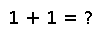scale

scale(A, b) scale(b, A)

Scale an array `A` by a scalar `b`, returning a new array.

If `A` is a matrix and `b` is a vector, then `scale(A,b)` scales each column `i` of `A` by `b[i]` (similar to `A*diagm(b)`), while `scale(b,A)` scales each row `i` of `A` by `b[i]` (similar to `diagm(b)*A`), returning a new array.

Note: for large `A`, `scale` can be much faster than `A .* b` or `b .* A`, due to the use of BLAS.

Examples

Checking you are not a robot: